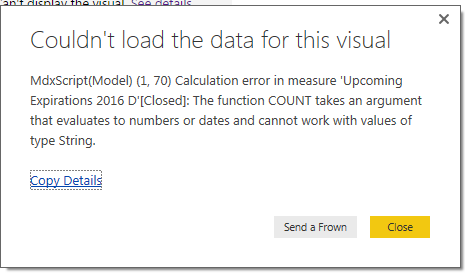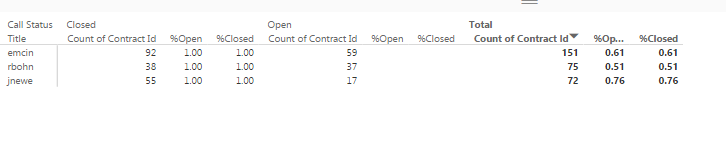cancel
Showing results for
Did you mean:Helper I

## Percent of open and closedI need to be able to have BI do what I've done in Excel its just a simple divide the open vs the closed for each person but I'm having issues how do that. The peoples name are the rows, and the call status is the column and the value is the count of the contract id. Thanks

Shawn

1 ACCEPTED SOLUTIONCommunity Champion

@ShawnPrice

Contract id is string?

If answer is yes, yo need to replace the measures:

TotalContracts=Calculate(Countrows(Table]))

Closed=Calculate(Countrows(Table]),Filter(Table,CallStatus="Close"))

Open=Calculate(Countrows(Table]),Filter(Table,CallStatus="Open"))

Lima - Peru
5 REPLIES 5Community Champion

@ShawnPrice

Hello:

Let's to try with these measures:

TotalContracts=Calculate(Count(Table[ContractId]))

Closed=Calculate(Count(Table[ContractId),Filter(Table,CallStatus="Close"))

Open=Calculate(Count(Table[ContractId),Filter(Table,CallStatus="Open"))

%Closed=Divide([Closed],[TotalContracts])

%Open=Divide([Open],[TotalContracts])

Lima - PeruHelper I

I have this done %Closed=Divide([Closed],[TotalContracts]) but I get this error now when I try to bring it on the page.Community Champion

@ShawnPrice

Contract id is string?

If answer is yes, yo need to replace the measures:

TotalContracts=Calculate(Countrows(Table]))

Closed=Calculate(Countrows(Table]),Filter(Table,CallStatus="Close"))

Open=Calculate(Countrows(Table]),Filter(Table,CallStatus="Open"))

Lima - PeruHelper I

So changing to count rows work, Thanks thats awesome. This is what I get now which is correct. Do you know if there is any good way to hide the extra columns that have showed up and just have the %Closed total at the end?Microsoft

Hi ShawnPrice,

Currently there is no option available to hide the extra columns, but there is a workaround which you may take a try:

"

hover your mouse over your column heading that you want to "hide" and move it to the right until the mouse cursor changes to a <->, then left-click and drag to the left until it is completely hidden.

"

How can i hide Column/measure in a Table

RegardsAnnouncements#### Exclusive opportunity for Women!

Join us for a free, hands-on Microsoft workshop led by women trainers for women where you will learn how to build a Dashboard in a Day!#### Power Platform Conference-Power BI and Fabric Sessions

Join us Oct 1 - 6 in Las Vegas for the Microsoft Power Platform Conference.Top Solution Authors
Top Kudoed Authors
Users online (1,625)